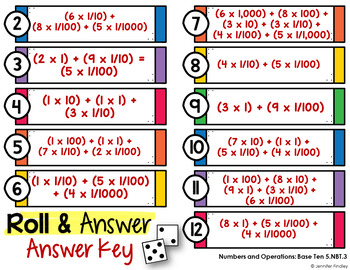DID YOU KNOW:
Seamlessly assign resources as digital activities

Learn how in 5 minutes with a tutorial resource. Try it Now

Learn More5th
Subjects
Standards
Resource Type
Formats Included
• PDF
•Google Apps™
Pages
13 (+ Answer Keys and Ink Saving Version)The Teacher-Author indicated this resource includes assets from Google Workspace (e.g. docs, slides, etc.).

#### Also included in

1. Math skill practice is still very much needed in classrooms. However, it doesn't have to be a stack of worksheets. It also doesn't always have to be a complicated, high-prep game. These Roll and Answer math activities for 5th grade are an easy way to spice up much-needed math practice.To complete th
\$10.00
\$16.00
Save \$6.00
2. Do you want your math stations and guided math centers done for the year? This 5th grade guided math bundle is the only resource you will need to have your math centers done for the year!Need Another Grade Level?Click here to see the 3rd Grade Guided Math Mega Bundle.Click here to see the 4th Grade
\$95.00
\$176.50
Save \$81.50

### Description

Math practice is still much needed in classrooms. However, it doesn't have to be stacks of worksheets. And it doesn't always have to be a complicated, high-prep game. These Roll and Answer math activities are an easy way to spice up much-needed math practice.

Put simply, the students roll two dice and add the amount shown. They find that amount on the page and solve that problem or answer that question.

You have the ability to keep them as simple as that or you can be more creative.

Here are two easy ideas for using these practice activities:

•Independent practice that is a bit more engaging than a worksheet

•Partner practice that allows students to work together to earn “points”

If you are familiar with BUMP and want a bit more gamification, you can use this as a BUMP game instead.

This specific set of Roll and Answer math centers includes 14 ready to use, self-checking math center games for the Numbers and Operations: Base Ten Common Core Standards for 5th Grade.

Here are the skills/activities included (one activity per skill):

1. Writing decimals represented by models
3. Multiplying by powers of 10
4. Dividing by powers of 10
5. Writing decimals in word form
6. Writing decimals in number form
7. Writing decimals in expanded form
8. Comparing decimals
9. Ordering decimals
10. Rounding decimals
11. Multi-digit multiplication
12. Multi-digit division
14. Multiplying and dividing decimals

The Roll and Answer math activities come in a printable and digital version.

Both versions can be completed independently or with partners, depending on your needs. Make sure you select the directions that you need.

The printable Roll and Answer math activities are included in color and an ink saving version.

The Roll and Answer math activities are also included in a digital version for use with Google Slides™.

The students use virtual dice (linked on the slides) to determine which question or task they will answer. A tutorial is included for students to help them use the virtual dice.

There are two options for directions: one for independent student use and one for partner use.

Digital recording sheets and self-checking answer keys are included. The self-checking answer keys can be deleted if you do not want the students to self- check their work.

The digital math activities can be used for math centers, digital math practice, partner activities, or even digital distance learning.

Please see the video preview to see how the digital math activities work.

Total Pages
13 (+ Answer Keys and Ink Saving Version)
Included
Teaching Duration
N/A
Report this Resource to TpT
Reported resources will be reviewed by our team. Report this resource to let us know if this resource violates TpT’s content guidelines.

### Standards

to see state-specific standards (only available in the US).
Add, subtract, multiply, and divide decimals to hundredths, using concrete models or drawings and strategies based on place value, properties of operations, and/or the relationship between addition and subtraction; relate the strategy to a written method and explain the reasoning used.
Find whole-number quotients of whole numbers with up to four-digit dividends and two-digit divisors, using strategies based on place value, the properties of operations, and/or the relationship between multiplication and division. Illustrate and explain the calculation by using equations, rectangular arrays, and/or area models.
Fluently multiply multi-digit whole numbers using the standard algorithm.
Use place value understanding to round decimals to any place.
Read, write, and compare decimals to thousandths.